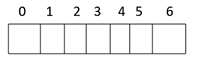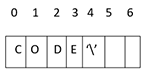# Strings in C ++

by Yogesh Khanna 11-Sep-18

0 553

As we have already studied in C language, there is a need for character array to handle strings. Let's start understanding string, write header files and then the main function.

For strings we have to write

char S; // array created in memory

and in this array, 7 values can be stored.

i.e.Now, if we want to store the value in it i.e. "CODE" the first character will go to the first block and automatically in remaining blocks '\0' will be stored.

char S = {'C', 'O', 'D', 'E','\0'};Illustrate with example

`#include <iostream>using namespace std;int main (){   char S = {'C', 'O', 'D', 'E',\0'};   cout << "lets start string: ";   cout << S << endl;   return 0;}`

Result:

lets start string: CODE

Another Basic Example of Strings

`#include <iostream>#include <string>using namespace std;int main (){  string mystring;  mystring = "This is basic example of string";  cout << mystring;  return 0;}`

Result:

This is a basic example of a string

In C language, strings are known as sequence of character but in the last character must be the null character. Otherwise, technically it is not a string. This feature of C is also adapted in C++.

If we wants to apply fundamental operators, the pre defined functions such as strcpy(), strcomp(), push_back(), getline(), pop_back() are required.

Share: